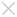### Suggestions

Home Page ZW3D Tips & Tricks Drawing Complex 2D and 3D Curves in ZW3D with Equations

## Learning

• ### Tips & Tricks

#### Drawing Complex 2D and 3D Curves in ZW3D with Equations

ZW3D from ZWSOF Tips an all-in-one CAD/CAM software program for designing consumer products, plastic molds, and CNC-machined parts. ZW3D has many functions for creating curves easily in 2D and 3D, such as lines, circles, arcs, ellipses, spirals, and splines. While these functions satisfy basic modeling needs, they do not make it easy to create curved shapes like 3D Archimedes spirals, butterfly curves, cap lines, and involutes.

To solve this problem, ZW3D includes the Equation Curve function. Its purpose is to generate curves from parametric equations that you define.Curves can be drawn in all of the coordinate systems supported by ZW3D, including rectangular, cylindrical, and spherical coordinate systems.

You define curves by choosing Equation Curve from the Insert menu.Notice the dialog box shown in figure 1.Figure 1: Equation curve editor

To how you how to use this function, follow the example I made of an involute curve. First, let me show you the pair of expressions needed to create involutes in Cartesian coordinates:

X=a (cost+tsint)

Y=a (sint-tcost )

where:

•  a is the radius of the circle

•  t is a parameter in the interval 0tpi.

ZW3D makes it easy to specifythe equations, as shown by “My involute” in figure 2. To add equations of your own to the list, enter them the X=, Y=, and Z= text entry boxes, and then click the Add into List--> button. (You can also update and remove functions from the list.) After entering your equation(s), click OK and ZW3D draws the curve based on the equations.Figure 2: “My involute” defines involute curves with two equations

In my example, the most important variable is t, because controls the variation of the curve. So, you can not use this variable name in equations.

The angle can be specified as radians or degrees under Units for Trigonometric Functions.It makes the equations short. You do not need to think about the 180° in the equation.

The Curve Variable option sets the quality of the curve. The larger the number of interpolate points, the smoother the curve.

And you can pick the point at which to insert the curve with the Pick AnotherInsert Point option. This point determines the point at which the curve is inserted into the drawing.

The result of my example curve appears as a feature in ZW3D,as shown below in figure 3:Figure 3: Result of plotting the involate curve

Please make sure the variables you set are the same as in the equations, and the equations must follow correct syntax. Then, the equation will run correctly; otherwise, it will show you the error message displayed by the dialog box in figure 4.Figure 4: Error message generated by equation with incorrect syntax

With this powerful function, you can to create the following types of curves easily. (See figure 5.)All you need to so is to double click the item names in the Equation List, and then click OK.Figure 5: 2D and 3D equations generated by ZW3D

While the curves in figure 5 look pretty, there are  also practical examples. Here, for example, is how I applied the equation curves toan industrial application:Figure 6: Spur gear and bevel wheel

Equations draw special cruves accurately, and help you make specific kinds of designs.

Now you know how to use the equation curves of ZW3D to work efficiently. If you come across any questions in using ZW3D, feel free to contact us at zw3d@zwsoft.com for help.

ZW3D is all-in-one, affordable CAD/CAM which enables concept to finished product design in an integrated, collaborative environment. The proprietary Overdrive kernel delivers 3D part and assembly modeling, 2D production drawings, reverse engineering, motion simulation, mold design and integrated CNC machining, simplifying the design process from concept to completion. Experience ZW3D 2012, where the only limit is your imagination. Please go to www.zwsoft.com to download a free 30-day trial today.

Short Description

Using parametric equations to generate complex curves ion 2D and 3D.

Keywords

http://www.zwsoft.com

Author’s Name:

Steven Sun

.

• Previous

• Next

## ·ZW3D 2016 Know-how:How to Use the Upgraded Pattern Command?2016-03-31